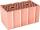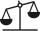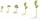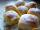# Pounds

3 pounds subtract 1/3 of a pound.

Result

x =  2.667

#### Solution:Leave us a comment of example and its solution (i.e. if it is still somewhat unclear...):

Showing 0 comments:Be the first to comment!#### To solve this example are needed these knowledge from mathematics:

Need help calculate sum, simplify or multiply fractions? Try our fraction calculator.

## Next similar examples:

1. 3 children3 children had to divide 4 pounds is candy. How much candy did each child get?
2. BrickBrick weight before drying is 5.3 kg. After drying, the bricks is 1 kg lighter and after firing is 0.7 kg lighter than after drying. What is the weight of the brick after drying and firing?
3. SchoolThere are 150 pupils in grade 5 . 2/3 of it are female. By what fractions are the males?
4. Flood waterFlood waters in some US village meant that the homes had to evacuate 364 people. 50 of them stayed at elementary schools, 59 them slept with their friends and others went to relatives. How many people have gone to relatives?
5. SubtractionTest what do you know about the subtraction of two numbers: a) make a difference if the minuend 4,307,288 and subtrahend is 472008 b) minuend is 4000 more than subtrahend. What's the difference? c) the difference is 38900 less than the minuend. What is th
6. BrickIsosceles scale has on one side all brick and second weight 1 kg and 1/4 of brick. The balance is in equilibrium. What is the weight of a brick?
7. SeedsOf the 72 wet seeds 7/8 germinated. How many seeds germinate?
8. Mixed2improperWrite the mixed number as an improper fraction. 166 2/3
9. Fraction to decimalWrite the fraction 3/22 as a decimal.
10. Fraction and a decimalWrite as a fraction and a decimal. One and two plus three and five hundredths
11. CakesOn the bowl were a few cakes. Jane ate one-third of them, Dana ate a quarter of those cakes that remained. a) What part (of the original number of cakes) Dana ate? b) At least how many cakes could be (initially) on thebowl?
12. Lengths of the poolMiguel swam 6 lengths of the pool. Mat swam 3 times as far as Miguel. Lionel swam 1/3 as far as Miguel. How many lengths did mat swim?
13. ZdeněkZdeněk picked up 15 l of water from a 100-liter full-water barrel. Write a fraction of what part of Zdeněk's water he picked.
14. In fractionsAn ant climbs 2/5 of the pole on the first hour and climbs 1/4 of the pole on the next hour. What part of the pole does the ant climb in two hours?
15. CookiesMom bake cookies. Rolo took 2/9 of all cookies, Michal 3/9. How many cookies ate Rolo if Michal had 9.
16. Jam cakesMom baked a third of plum jam cakes, one third cheesecakes and 18 poppy. How many cakes she had bake?
17. Addition of Roman numbersAdded together and write as decimal number: LXVII + MLXIV